# Impulse Formula

Impulse Formula

Impulse is a quantity that is closely related to momentum. Impulse is a vector, with both a value and a direction, and is represented by the symbol. Impulse is the product of a constant force, and a time interval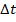. The Greek letter("delta") is used to mean "the change in", andis the amount of time for which the force is applied. The unit of impulse is the Newton-second,.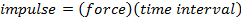= impulse ()= applied force (N)= time interval for which the force is applied (s)

Impulse Formula Questions:

1) A thruster rocket on a spaceship exerts a force of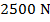, in the starboard direction. If the thruster is on for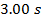, what is the resulting impulse?

Answer: The direction of the impulse will be the same as the applied force (starboard direction). The magnitude of the impulse can be found using the formula: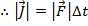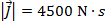The impulse resulting from the thruster rocket is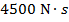, in the starboard direction.

2) A baseball player hits a ball with the bat, exerting a force with a magnitude of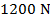. If the resulting impulse has a magnitude of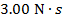, how long were the ball and bat in contact with each other?

Answer: The direction of the force and the impulse will be the same, though this problem only involves the magnitudes of these quantities. The amount of time that the ball and bat were in contact can be found by rearranging the impulse formula: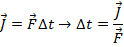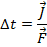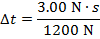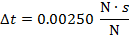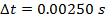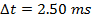The bat and ball were only in contact for, or(milli-seconds).